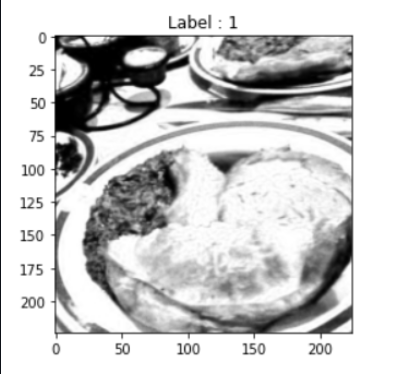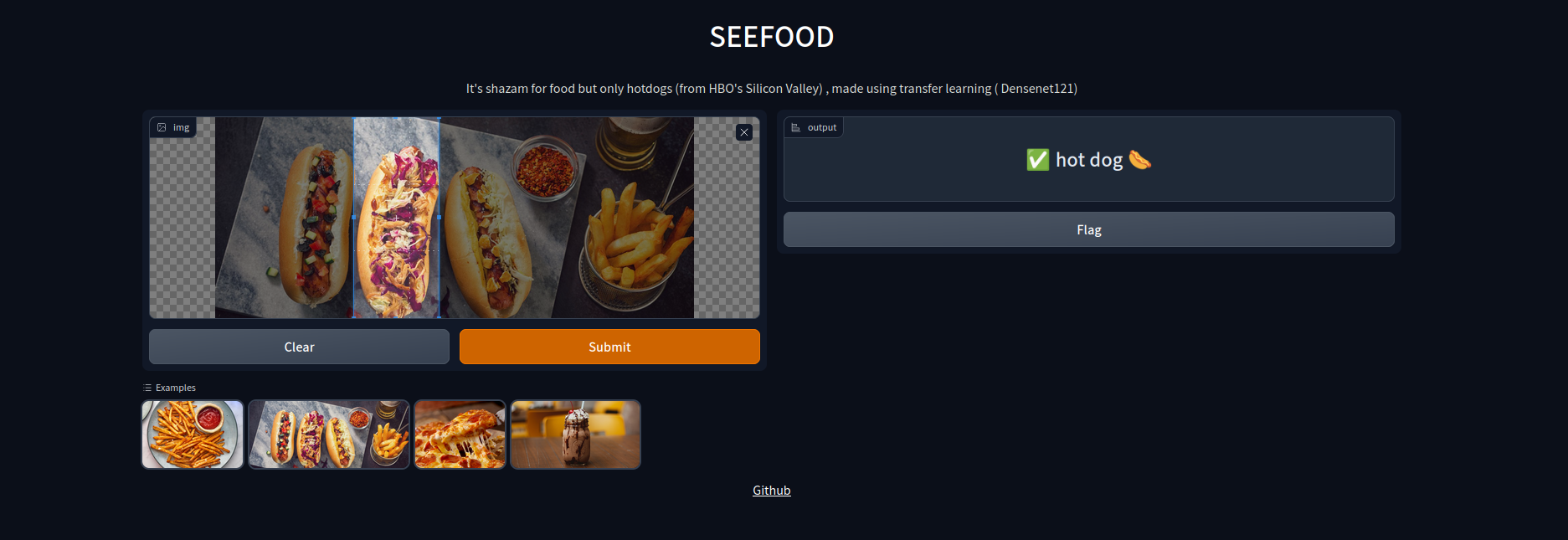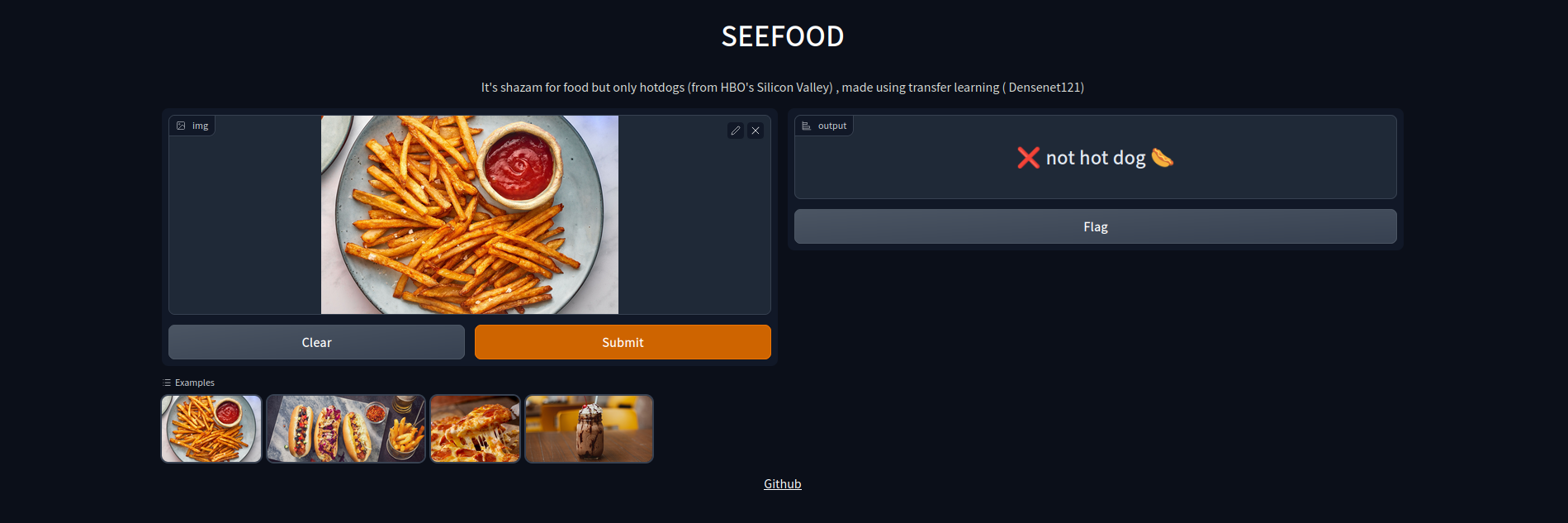SEEFOOD is an application which basically predicts if an image is an image of a hot-dog or not. This is based on the model used in the HBO’s Silicon Valley .This blog is a short tutorial on building this classfier model. Knowing the basics of Deep Learning, CNNs and Transfer learning would be helpful in following along.### How to start ?

First, we need to get a dataset for training the model. For this, there is a dataset on kaggle which perfectly matches our needs. So now that we have a dataset, we need to build and train the actual model.

### Let’s start coding.

The notebook is here if you want to follow through as you’re reading the article.

First, off we import all the required libraries.

``````
import torch
from torchvision import datasets,transforms, models
from torch import optim , nn
import torch.nn.functional as F
import matplotlib.pyplot as plt
``````

Before importing the dataset we can use a simple transform to ensure all our images and resized, center cropped, and convert into tensors.

``````transforms = transforms.Compose([transforms.Resize(256),
transforms.CenterCrop(224),
transforms.ToTensor(),])

dataset = datasets.ImageFolder(root='gdrive/My Drive/seefood/train/',transform=transforms)
validation_set = datasets.ImageFolder(root='gdrive/My Drive/seefood/test/',transform=transforms)
``````

Now we can import both the test and validation sets. Since the number of images here is not very high we can use a batch size of 20.

Once the dataset is loaded we can view the images.

``````trainLoader = DataLoader(dataset, batch_size=20,shuffle= True)

images ,labels = next(data)
plt.imshow(images[0,:,:],cmap='gray')
plt.title(f"Label : {labels}")
``````### Building the model

Since we are dealing with images we would need to use a model which is based on Convolutional Neural Networks (CNNs). The model itself would be a binary classifier to detect whether the food item is a “hot dog” or not. Smaller models with lower layers do not tend to work because they are simply not powerful enough to detect the different features, so we can see a good use case of transfer learning here.

For this purpose we can use the densenet121 model, you can read more about Densely Connected Convolutional Networks in this paper here. . Since we are only going to train the last block of the model we can freeze all the other parameters of the model.

``````model = models.densenet121(pretrained=True)
for params in model.parameters():
#model.classifier -> Linear(in_features=1024, out_features=1000, bias=True)
``````

If we take a look at the classifier currently we can see it’s a classifier that has 1024 input features and 1000 output features. We can redefine this classifier for our case here.

``````classifier = nn.Sequential(nn.Linear(1024,1024),nn.ReLU(),nn.Dropout(p=0.3),
nn.Linear(1024,512),nn.ReLU(),nn.Dropout(p=0.3),
nn.Linear(512,2),nn.LogSoftmax(dim=1))
model.classifier = classifier

``````

The classifier now,

``````model.classifier -> Sequential(
(0): Linear(in_features=1024, out_features=1024, bias=True)
(1): ReLU()
(2): Dropout(p=0.3, inplace=False)
(3): Linear(in_features=1024, out_features=512, bias=True)
(4): ReLU()
(5): Dropout(p=0.3, inplace=False)
(6): Linear(in_features=512, out_features=2, bias=True)
(7): LogSoftmax(dim=1)
)
``````

### Training the model

For the loss function here we can use the negative log-likelihood loss. Since we are training only the classifier part of the model, we need to include only the parameters from that block in the optimizer.

``````loss_function = nn.NLLLoss()
``````

If you are trying to train the model, do make use of GPUs on google colab or kaggle. This speeds up the training process a lot. The standard training loop,

``````loss_graph , val_loss_graph , acc = [] , [] , []
for _ in range(6):
running_loss = 0
val_loss = 0
device = torch.device("cuda" if torch.cuda.is_available() else "cpu")
model.train()

images , labels = images.to(device), labels.to(device)
logits = model(images)
loss = loss_function(logits,labels )
running_loss += loss.item()
loss.backward()

optimizer.step()

model.eval()
cor = 0
total = 0

images , labels = images.to(device), labels.to(device)
predictions = model(images)
loss = loss_function(predictions, labels)
val_loss += loss.item()

for p,l in zip(torch.argmax(predictions,dim=1 ),labels):
if p==l:
cor +=1
total +=1

acc.append(cor*100/total)
print(f'training loss : {running_loss/len(trainLoader)} , validation loss : {val_loss/len(valLoader)} , Accuracy : {cor*100/total}')
``````

```training loss : 0.8344280552864075 , validation loss : 0.38366479575634005 , Accuracy : 87.4 training loss : 0.3701903349161148 , validation loss : 0.4923225581645966 , Accuracy : 76.2 training loss : 0.40178473711013796 , validation loss : 0.26429639220237733 , Accuracy : 90.2 training loss : 0.29359916508197786 , validation loss : 0.2639751332998276 , Accuracy : 89.6 training loss : 0.23448901653289794 , validation loss : 0.26386004567146304 , Accuracy : 89.6 ```

### Result

On training it for about 6 epochs I got around 86% accuracy. You can try this here### Interesting question ?

Why do you think densenet121 was used for this model ?